Trang chủ‎ > ‎IT‎ > ‎Machine Learning‎ > ‎

## Introduction

Too much of anything is good for nothing!

What happens when a data set has too many variables ? Here are few possible situations which you might come across:

1. You find that most of the variables are correlated.
2. You lose patience and decide to run a model on whole data. This returns poor accuracy and you feel terrible.
3. You become indecisive about what to do
4. You start thinking of some strategic method to find few important variables

Trust me, dealing with such situations isn’t as difficult as it sounds. Statistical techniques such as factor analysis, principal component analysis help to overcome such difficulties.

In this post, I’ve explained the concept of principal component analysis in detail. I’ve kept the explanation to be simple and informative. For practical understanding, I’ve also demonstrated using this technique in R with interpretations.

Note: Understanding this concept requires prior knowledge of statistics.

Update (as on 28th July): Process of Predictive Modeling with PCA Components in R is added below.## What is Principal Component Analysis ?

In simple words, principal component analysis is a method of extracting important variables (in form of components) from a large set of variables available in a data set. It extracts low dimensional set of features from a high dimensional data set with a motive to capture as much information as possible. With fewer variables, visualization also becomes much more meaningful. PCA is more useful when dealing with 3 or higher dimensional data.

It is always performed on a symmetric correlation or covariance matrix. This means the matrix should be numeric and have standardized data.

Let’s understand it using an example:

Let’s say we have a data set of dimension 300 (n) × 50 (p). n represents the number of observations and p represents number of predictors. Since we have a large p = 50, there can be `p(p-1)/2` scatter plots i.e more than 1000 plots possible to analyze the variable relationship. Wouldn’t is be a tedious job to perform exploratory analysis on this data ?

In this case, it would be a lucid approach to select a subset of p (p << 50) predictor which captures as much information. Followed by plotting the observation in the resultant low dimensional space.

The image below shows the transformation of a high dimensional data (3 dimension) to low dimensional data (2 dimension) using PCA. Not to forget, each resultant dimension is a linear combination of p features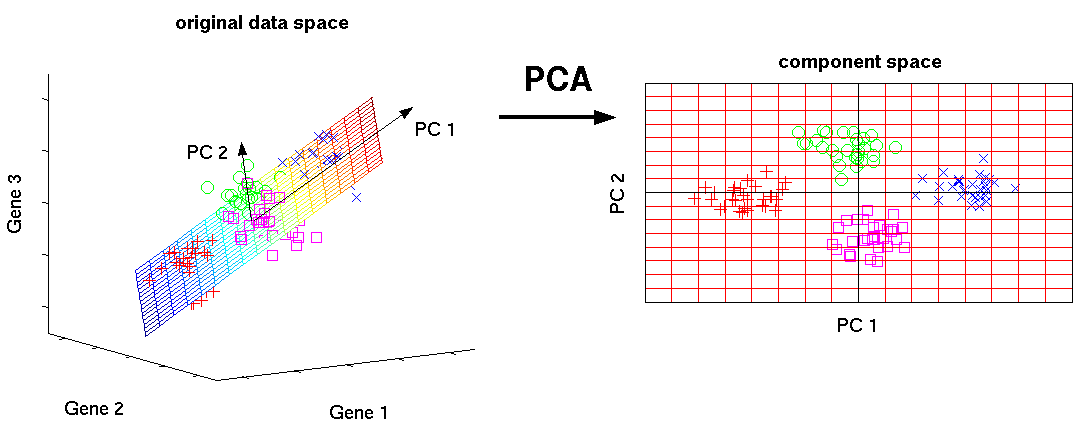Source: nlpca

## What are principal components ?

A principal component is a normalized linear combination of the original predictors in a data set. In image above, PC1 and PC2 are the principal components. Let’s say we have a set of predictors as`X¹, X²...,Xp`

The principal component can be written as:

`Z¹ = Φ¹¹X¹ + Φ²¹X² + Φ³¹X³ + .... +`Φp`¹Xp`

where,

• Z¹ is first principal component
• `Φp¹` is the loading vector comprising of loadings (`Φ¹, Φ²..`) of first principal component. The loadings are constrained to a sum of square equals to 1. This is because large magnitude of loadings may lead to large variance. It also defines the direction of the principal component (Z¹) along which data varies the most. It results in a line in p dimensional space which is closest to the n observations. Closeness is measured using average squared euclidean distance.
• `X¹..Xp` are normalized predictors. Normalized predictors have mean equals to zero and standard deviation equals to one.

Therefore,

First principal component is a linear combination of original predictor variables which captures the maximum variance in the data set. It determines the direction of highest variability in the data. Larger the variability captured in first component, larger the information captured by component. No other component can have variability higher than first principal component.

The first principal component results in a line which is closest to the data i.e. it minimizes the sum of squared distance between a data point and the line.

Similarly, we can compute the second principal component also.

Second principal component (`Z²`) is also a linear combination of original predictors which captures the remaining variance in the data set and is uncorrelated with `Z¹`. In other words, the correlation between first and second component should is zero. It can be represented as:

`Z² = Φ¹²X¹ + Φ²²X² + Φ³²X³ + .... + Φp2Xp`

If the two components are uncorrelated, their directions should be orthogonal (image below). This image is based on a simulated data with 2 predictors. Notice the direction of the components, as expected they are orthogonal. This suggests the correlation b/w these components in zero.All succeeding principal component follows a similar concept i.e. they capture the remaining variation without being correlated with the previous component. In general, for n × p dimensional data, min(n-1, p) principal component can be constructed.

The directions of these components are identified in an unsupervised way i.e. the response variable(Y) is not used to determine the component direction. Therefore, it is an unsupervised approach.

Note: Partial least square (PLS) is a supervised alternative to PCA. PLS assigns higher weight to variables which are strongly related to response variable to determine principal components.

## Why is normalization of variables necessary ?

The principal components are supplied with normalized version of original predictors. This is because, the original predictors may have different scales. For example: Imagine a data set with variables’ measuring units as gallons, kilometers, light years etc. It is definite that the scale of variances in these variables will be large.

Performing PCA on un-normalized variables will lead to insanely large loadings for variables with high variance. In turn, this will lead to dependence of a principal component on the variable with high variance. This is undesirable.

As shown in image below, PCA was run on a data set twice (with unscaled and scaled predictors). This data set has ~40 variables. You can see, first principal component is dominated by a variable Item_MRP. And, second principal component is dominated by a variable Item_Weight. This domination prevails due to high value of variance associated with a variable. When the variables are scaled, we get a much better representation of variables in 2D space.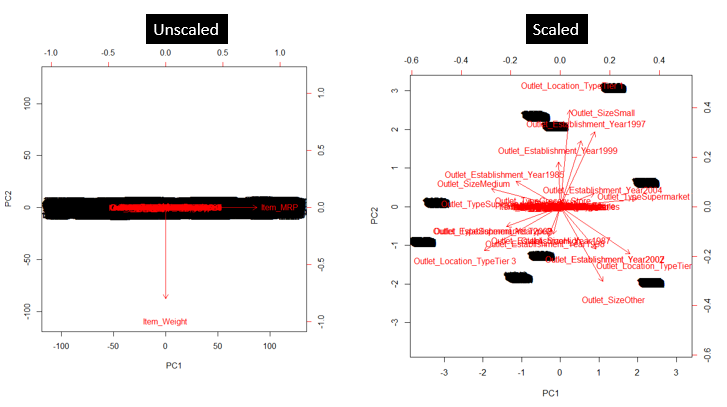## Implement PCA in R & Python (with interpretation)

How many principal components to choose ? I could dive deep in theory, but it would be better to answer these question practically.

For this demonstration, I’ll be using the data set from Big Mart Prediction Challenge III.

Remember, PCA can be applied only on numerical data. Therefore, if the data has categorical variables they must be converted to numerical. Also, make sure you have done the basic data cleaning prior to implementing this technique. Let’s quickly finish with initial data loading and cleaning steps:

`#directory path`
`> path <- ".../Data/Big_Mart_Sales"`

`#set working directory`
`> setwd(path)`

`#load train and test file`
`> train <- read.csv("train_Big.csv")`
`> test <- read.csv("test_Big.csv")`

`#add a column`
`> test\$Item_Outlet_Sales <- 1`

`#combine the data set`
`> combi <- rbind(train, test)`

`#impute missing values with median`
`> combi\$Item_Weight[is.na(combi\$Item_Weight)] <- median(combi\$Item_Weight, na.rm = TRUE)`

`#impute 0 with median`
`> combi\$Item_Visibility <- ifelse(combi\$Item_Visibility == 0, median(combi\$Item_Visibility),                                   combi\$Item_Visibility)`

`#find mode and impute`
`> table(combi\$Outlet_Size, combi\$Outlet_Type)`
`> levels(combi\$Outlet_Size) <- "Other"`

Till here, we’ve imputed missing values. Now we are left with removing the dependent (response) variable and other identifier variables( if any). As we said above, we are practicing an unsupervised learning technique, hence response variable must be removed.

`#remove the dependent and identifier variables`
`> my_data <- subset(combi, select = -c(Item_Outlet_Sales, Item_Identifier,                                       Outlet_Identifier))`

Let’s check the available variables ( a.k.a predictors) in the data set.

`#check available variables`
`> colnames(my_data)`

Since PCA works on numeric variables, let’s see if we have any variable other than numeric.

`#check variable class`
`> str(my_data)`

`'data.frame': 14204 obs. of 9 variables:`
`\$ Item_Weight : num 9.3 5.92 17.5 19.2 8.93 ...`
`\$ Item_Fat_Content : Factor w/ 5 levels "LF","low fat",..: 3 5 3 5 3 5 5 3 5 5 ...`
`\$ Item_Visibility : num 0.016 0.0193 0.0168 0.054 0.054 ...`
`\$ Item_Type : Factor w/ 16 levels "Baking Goods",..: 5 15 11 7 10 1 14 14 6 6 ...`
`\$ Item_MRP : num 249.8 48.3 141.6 182.1 53.9 ...`
`\$ Outlet_Establishment_Year: int 1999 2009 1999 1998 1987 2009 1987 1985 2002 2007 ...`
`\$ Outlet_Size : Factor w/ 4 levels "Other","High",..: 3 3 3 1 2 3 2 3 1 1 ...`
`\$ Outlet_Location_Type : Factor w/ 3 levels "Tier 1","Tier 2",..: 1 3 1 3 3 3 3 3 2 2 ...`
`\$ Outlet_Type : Factor w/ 4 levels "Grocery Store",..: 2 3 2 1 2 3 2 4 2 2 ...`

Sadly, 6 out of 9 variables are categorical in nature. We have some additional work to do now. We’ll convert these categorical variables into numeric using one hot encoding.

`#load library`
`> library(dummies)`

`#create a dummy data frame`
`> new_my_data <- dummy.data.frame(my_data, names = c("Item_Fat_Content","Item_Type",`
`                                "Outlet_Establishment_Year","Outlet_Size",`
`                                "Outlet_Location_Type","Outlet_Type"))`

To check, if we now have a data set of integer values, simple write:

`#check the data set`
`> str(new_my_data)`

And, we now have all the numerical values. Let’s divide the data into test and train.

`#divide the new data`
`> pca.train <- new_my_data[1:nrow(train),]`
`> pca.test <- new_my_data[-(1:nrow(train)),]`

We can now go ahead with PCA.

The base R function prcomp() is used to perform PCA. By default, it centers the variable to have mean equals to zero. With parameter `scale. = T`, we normalize the variables to have standard deviation equals to 1.

`#principal component analysis`
`> prin_comp <- prcomp(pca.train, scale. = T)`
`> names(prin_comp)`
` "sdev"     "rotation" "center"   "scale"    "x"`

The prcomp() function results in 5 useful measures:

1. center and scale refers to respective mean and standard deviation of the variables that are used for normalization prior to implementing PCA

`#outputs the mean of variables`
`prin_comp\$center`

`#outputs the standard deviation of variables`
`prin_comp\$scale`

2. The rotation measure provides the principal component loading. Each column of rotation matrix contains the principal component loading vector. This is the most important measure we should be interested in.

`> prin_comp\$rotation`

This returns 44 principal components loadings. Is that correct ? Absolutely. In a data set, the maximum number of principal component loadings is a minimum of (n-1, p). Let’s look at first 4 principal components and first 5 rows.

`> prin_comp\$rotation[1:5,1:4]`
`                                PC1            PC2            PC3             PC4`
`Item_Weight                0.0054429225   -0.001285666   0.011246194   0.011887106`
`Item_Fat_ContentLF        -0.0021983314    0.003768557  -0.009790094  -0.016789483`
`Item_Fat_Contentlow fat   -0.0019042710    0.001866905  -0.003066415  -0.018396143`
`Item_Fat_ContentLow Fat    0.0027936467   -0.002234328   0.028309811   0.056822747`
`Item_Fat_Contentreg        0.0002936319    0.001120931   0.009033254  -0.001026615`

3. In order to compute the principal component score vector, we don’t need to multiply the loading with data. Rather, the matrix x has the principal component score vectors in a 8523 × 44 dimension.

> dim(prin_comp\$x)
 8523    44

Let’s plot the resultant principal components.

`> biplot(prin_comp, scale = 0)`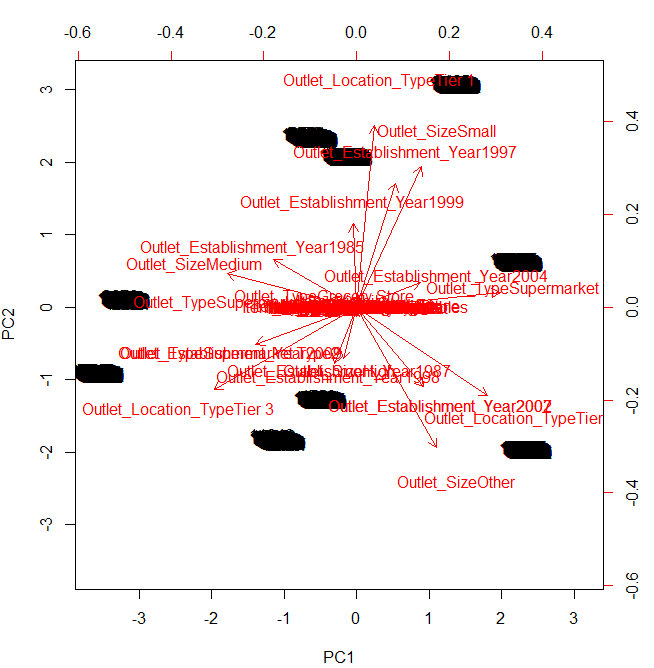The parameter `scale = 0` ensures that arrows are scaled to represent the loadings. To make inference from image above, focus on the extreme ends (top, bottom, left, right) of this graph.

We infer than first principal component corresponds to a measure of Outlet_TypeSupermarket, Outlet_Establishment_Year 2007. Similarly, it can be said that the second component corresponds to a measure of Outlet_Location_TypeTier1, Outlet_Sizeother. For exact measure of a variable in a component, you should look at rotation matrix(above) again.

4. The prcomp() function also provides the facility to compute standard deviation of each principal component. sdev refers to the standard deviation of principal components.

`#compute standard deviation of each principal component`
`> std_dev <- prin_comp\$sdev`

`#compute variance`
`> pr_var <- std_dev^2`

`#check variance of first 10 components`
`> pr_var[1:10]`
` 4.563615 3.217702 2.744726 2.541091 2.198152 2.015320 1.932076 1.256831`
` 1.203791 1.168101`

We aim to find the components which explain the maximum variance. This is because, we want to retain as much information as possible using these components. So, higher is the explained variance, higher will be the information contained in those components.

To compute the proportion of variance explained by each component, we simply divide the variance by sum of total variance. This results in:

`#proportion of variance explained`
`> prop_varex <- pr_var/sum(pr_var)`
`> prop_varex[1:20]`
` 0.10371853 0.07312958 0.06238014 0.05775207 0.04995800 0.04580274`
` 0.04391081 0.02856433 0.02735888 0.02654774 0.02559876 0.02556797`
` 0.02549516 0.02508831 0.02493932 0.02490938 0.02468313 0.02446016`
` 0.02390367 0.02371118`

This shows that first principal component explains 10.3% variance. Second component explains 7.3% variance. Third component explains 6.2% variance and so on. So, how do we decide how many components should we select for modeling stage ?

The answer to this question is provided by a scree plot. A scree plot is used to access components or factors which explains the most of variability in the data. It represents values in descending order.

`#scree plot`
`> plot(prop_varex, xlab = "Principal Component",`
`             ylab = "Proportion of Variance Explained",`
`             type = "b")`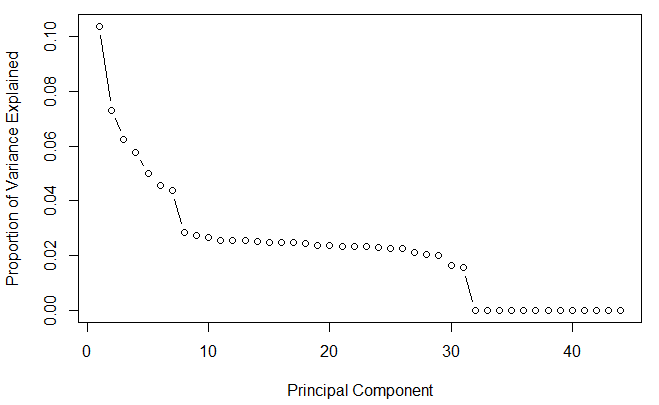The plot above shows that ~ 30 components explains around 98.4% variance in the data set. In order words, using PCA we have reduced 44 predictors to 30 without compromising on explained variance. This is the power of PCA> Let’s do a confirmation check, by plotting a cumulative variance plot. This will give us a clear picture of number of components.

`#cumulative scree plot`
`> plot(cumsum(prop_varex), xlab = "Principal Component",`
`              ylab = "Cumulative Proportion of Variance Explained",`
`              type = "b")`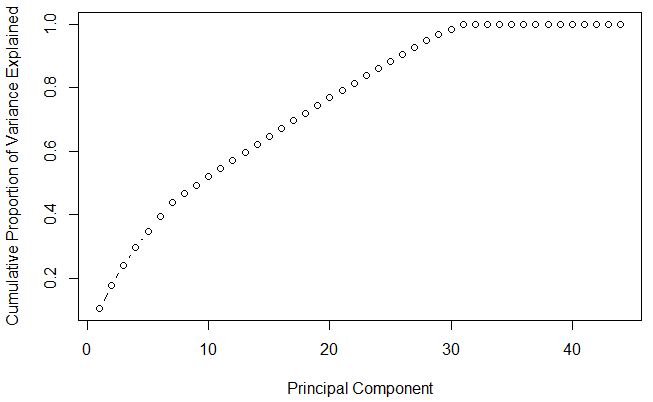This plot shows that 30 components results in variance close to ~ 98%. Therefore, in this case, we’ll select number of components as 30 [PC1 to PC30] and proceed to the modeling stage. This completes the steps to implement PCA on train data. For modeling, we’ll use these 30 components as predictor variables and follow the normal procedures.

### Predictive Modeling with PCA Components

After we’ve calculated the principal components on training set, let’s now understand the process of predicting on test data using these components. The process is simple. Just like we’ve obtained PCA components on training set, we’ll get another bunch of components on testing set. Finally, we train the model.

But, few important points to understand:

1. We should not combine the train and test set to obtain PCA components of whole data at once. Because, this would violate the entire assumption of generalization since test data would get ‘leaked’ into the training set. In other words, the test data set would no longer remain ‘unseen’. Eventually, this will hammer down the generalization capability of the model.
2. We should not perform PCA on test and train data sets separately. Because, the resultant vectors from train and test PCAs will have different directions ( due to unequal variance). Due to this, we’ll end up comparing data registered on different axes. Therefore, the resulting vectors from train and test data should have same axes.

So, what should we do?

We should do exactly the same transformation to the test set as we did to training set, including the center and scaling feature. Let’s do it in R:

`#add a training set with principal components`
`> train.data <- data.frame(Item_Outlet_Sales = train\$Item_Outlet_Sales, prin_comp\$x)`

`#we are interested in first 30 PCAs`
`> train.data <- train.data[,1:31]`

`#run a decision tree`
`> install.packages("rpart")`
`> library(rpart)`
`> rpart.model <- rpart(Item_Outlet_Sales ~ .,data = train.data, method = "anova")`
`> rpart.model`

`#transform test into PCA`
`> test.data <- predict(prin_comp, newdata = pca.test)`
`> test.data <- as.data.frame(test.data)`

`#select the first 30 components`
`> test.data <- test.data[,1:30]`

`#make prediction on test data`
`> rpart.prediction <- predict(rpart.model, test.data)`

`#For fun, finally check your score of leaderboard`
`> sample <- read.csv("SampleSubmission_TmnO39y.csv")`
`> final.sub <- data.frame(Item_Identifier = sample\$Item_Identifier, Outlet_Identifier = sample\$Outlet_Identifier, Item_Outlet_Sales = rpart.prediction)`
`> write.csv(final.sub, "pca.csv",row.names = F)`

That’s the complete modeling process after PCA extraction. I’m sure you wouldn’t be happy with your leaderboard rank after you upload the solution. Try using random forest!

For Python Users: To implement PCA in python, simply import PCA from sklearn library. The interpretation remains same as explained for R users above. Ofcourse, the result is some as derived after using R. The data set used for Python is a cleaned version where missing values have been imputed, and categorical variables are converted into numeric. The modeling process remains same, as explained for R users above.

`import numpy as np`
`from sklearn.decomposition import PCA`
`import pandas as pd`
`import matplotlib.pyplot as plt`
`from sklearn.preprocessing import scale`
`%matplotlib inline`

`#Load data set`
`data = pd.read_csv('Big_Mart_PCA.csv')`

`#convert it to numpy arrays`
`X=data.values`

`#Scaling the values`
`X = scale(X)`

`pca = PCA(n_components=44)`

`pca.fit(X)`

`#The amount of variance that each PC explains`
`var= pca.explained_variance_ratio_`

`#Cumulative Variance explains`
`var1=np.cumsum(np.round(pca.explained_variance_ratio_, decimals=4)*100)`

`print var1`
`[  10.37   17.68   23.92   29.7    34.7    39.28   43.67   46.53   49.27`
`51.92   54.48   57.04   59.59   62.1    64.59   67.08   69.55   72.`
`74.39   76.76   79.1    81.44   83.77   86.06   88.33   90.59   92.7`
`94.76   96.78   98.44  100.01  100.01  100.01  100.01  100.01  100.01`
`100.01  100.01  100.01  100.01  100.01  100.01  100.01  100.01]`

`plt.plot(var1`)`#Looking at above plot I'm taking 30 variables`
`pca = PCA(n_components=30)`
`pca.fit(X)`
`X1=pca.fit_transform(X)`

`print X1`

## Points to Remember

1. PCA is used to overcome features redundancy in a data set.
2. These features are low dimensional in nature.
3. These features a.k.a components are a resultant of normalized linear combination of original predictor variables.
4. These components aim to capture as much information as possible with high explained variance.
5. The first component has the highest variance followed by second, third and so on.
6. The components must be uncorrelated (remember orthogonal direction ? ). See above.
7. Normalizing data becomes extremely important when the predictors are measured in different units.
8. PCA works best on data set having 3 or higher dimensions. Because, with higher dimensions, it becomes increasingly difficult to make interpretations from the resultant cloud of data.
9. PCA is applied on a data set with numeric variables.
10. PCA is a tool which helps to produce better visualizations of high dimensional data.

## End Notes

This brings me to the end of this tutorial. Without delving deep into mathematics, I’ve tried to make you familiar with most important concepts required to use this technique. It’s simple but needs special attention while deciding the number of components.  Practically, we should strive to retain only first few k components

The idea behind pca is to construct some principal components( Z << Xp ) which satisfactorily explains most of the variability in the data, as well as relationship with the response variable.